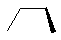# Conformational Energy

## Objective

The objective of this exercise is to calculate the strain energy incurred as a molecule moves between various conformations.

## Procedure

Use PM3, semi-empirical, calculations to calculate the strain energy of the following molecules. The strain energy can be calculated by subtracting the heat of formation of the more stable species from that of the less stable species. The strain energy should be a positive quantity.

 Molecule Strain Energy (kJ/mol)02.17017.1